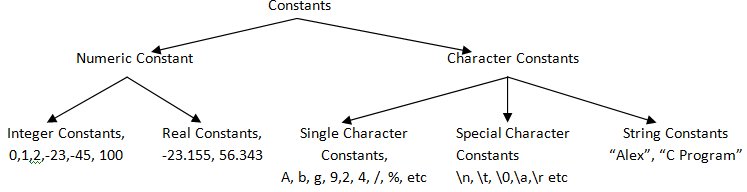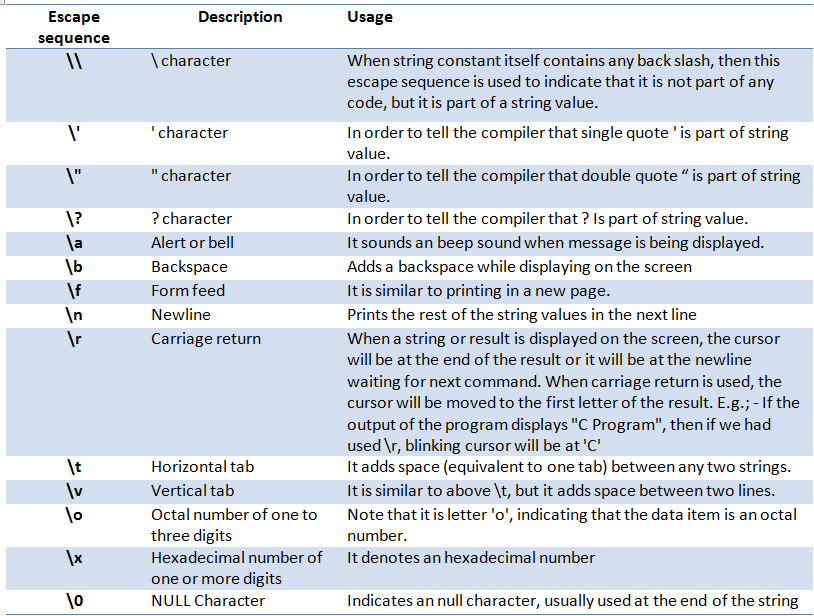# Constants in C Programming

Constants are also variables but their values will not change throughout the program. They are also called as literals. They can be integer constants, floating constant or string constants.Integer constants are the constant variables which hold only integer values – only whole numbers.  Following rules needs to be kept in mind while creating a integer constant values.

• It should have at least one digit.
• It should not have any decimal numbers.
• It can be positive number or negative number or zero.
• It cannot have comma or blank spaces in it.
• It can have values ranging from  -32768 to 32767
• It can have decimal numbers, octal numbers or hexadecimal numbers.

E.g.: –    Decimal constants:  0,1, 23, 4546, 676, -10, -1023 etc
Octal constants: 021, 123, 033, 2467 etc
Hexadecimal constants: 0x7f, 0x2a, 0x34acd etc

Floating / Real constants are the constants which hold only fractional numbers.

• It should have at least one digit.
• It must have a decimal point.
• It can either be positive or negative number.
• It cannot have commas or blanks are allowed.
• It can be in exponential form – with mantissa part and an exponential part which is separated by a letter ‘e’.
• Both mantissa and exponential part can be positive or negative numbers.
• There should be at least one digit in the exponential part.
• Floating Values can range from -3.4e38 and +3.4e38

E.g.: – 0.2334, 45.565, 2334.54456, -0.2323, -0.562E-5

Character constant will have single constant character values.

• It can be an alphabet, a single digit or a single special symbol enclosed within inverted commas (‘ and ‘).
• It can have only 1 character and allocates only 1 byte of memory.

E.g.: – A, F, g, I, z, Z, v etc

String constant will have constant string values.

• It can be combination of alphabet, a single digit or a single special symbol enclosed within double inverted commas (“ and “).
• It can have as many characters as we want and allocate memory equal to number of character bytes.

E.g.: – “C Program”, “String Constant Example!”, “C”, “ “ etc.

We can have special character constants too. They are usually referred as escape sequence. Most frequently used escape sequence and their usages are listed below.Translate »